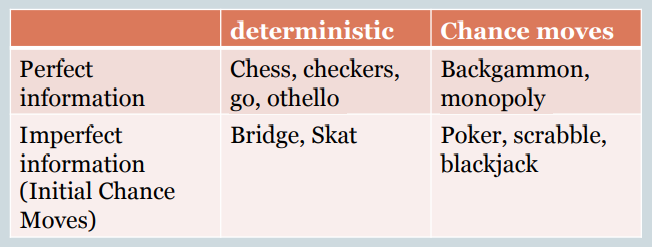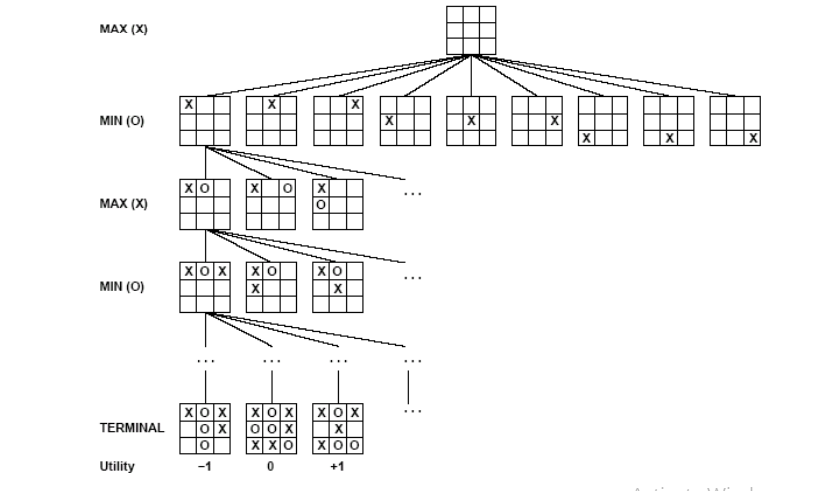# Python Learning Week 11

Mini Project: Adversarial Search or Playing Games with Python

Game name: Tic Tac Toe (Using: The Minimax Algorithm)

• Examine the problems that arise when we try to plan ahead in a world where other agents are planning against us.
•  A good example is in board games.
• Adversarial games, while much studied in AI, are a small part of game theory in economics.
Typical AI assumptions
• Two agents whose actions alternate
• Utility values for each agent are the opposite of the
other
• Fully observable environments
• In game theory terms: Zero-sum games of perfect
information.
• We’ll relax these assumptions later.

Search versus Games

• Solution is (heuristic) method for finding goal
• Heuristic techniques can find optimal solution
• Evaluation function: estimate of cost from start to goal through given node
• Examples: path planning, scheduling activities
• Solution is strategy (strategy specifies move for every possible opponent
• Optimality depends on opponent. Why?
•  Time limits force an approximate solution
• Evaluation function: evaluate “goodness” of game position
• Examples: chess, checkers, Othello, backgammon

Types of GamesGame Setup

1. Two players: MAX and MIN

2. MAX moves first and they take turns until the game is over

• Winner gets award, loser gets penalty.

3. Games as search:

• Initial state: e.g. board configuration of chess
• Successor function: list of (move, state) pairs specifying legal moves.
•  Terminal test: Is the game finished?
•  Utility function: Gives numerical value of terminal states. E.g. win (+1), lose
(-1) and draw (0) in tic-tac-toe or chess

4. MAX uses search tree to determine next move.

Size of search trees

1. b = branching factor
2. d = number of moves by both players
3. Search tree is O(bd)
Chess
1. b ~ 35
2. D ~100
3. – search tree is ~ 10 154 (!!)
4. – completely impractical to search this
Game-playing emphasizes being able to make optimal decisions in a finite amount of time
1. Somewhat realistic as a model of a real-world agent
2. Even if games themselves are artificial

Partial Game Tree for Tic-Tac-Toe